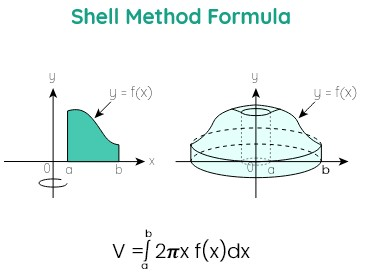# Shell Method Calculator

To find the volume, Enter the function, select the variable, enter the limit values, and click calculate button using shell method calculatorThis will be calculated:

Give Us Feedback

## Shell Method Calculator

Shells method calculator is used to find the volume and surface area of the given function. This shell calculator solves the definite integral of the function by applying the upper and lower limit value of the function.

It provides the solution with steps of the given function.

## What is shell method?

In mathematics, the shell method is a technique of determining volumes by decomposing a solid of revolution into cylindrical shells. It is the alternate way of wisher method. The volume of the cylinder is usually equal to the πr2h.## Formulas of shell method

There are different kinds of formulas of shell method depending on the axis of curves.

Revolving the area under the curve of f(x).

Volume = V = 2π $\int _a^b\:$x f(x) dx

Revolving the area under the curve of f(y).

Volume = V = 2π $\int _a^b\:$y f(y) dy

1. ### Between two curves about y-axis

Revolving the area between two curves f(x) and g(x)

Volume = V = 2π $\int _a^b\:$x[f(x) – g(x)] dx

1. ### Between two curves about x-axis

Revolving the area between two curves f(y) and g(y)

Volume = V = 2π $\int _a^b\:$y[f(y) – g(y)] dy

1. ### Between two curves about x = h

Revolving the area between two curves f(x) and g(x)

Volume = V = 2π $\int _a^b\:$(x – h) [f(x) – g(x)] dx

1. ### Between two curves about y = k

Revolving the area between two curves f(y) and g(y)

Volume = V = 2π $\int _a^b\:$(y – k) [f(y) – g(y)] dy

The above six formulas are used to solve the problems of the shell method in different scenarios.

## How to calculate the shell method?

Below are a few solved examples of the shell method.

Example 1:

Calculate the shell method about y-axis if f(x) = 6x2 + 4 and the interval is {2, 3}.

Solution

Step 1: Take the given information.

f(x) = 6x2 + 4

Lower limit = a = 2

Upper limit = b = 3

Step 2: Take the formula of the shell method about y-axis.

Volume = V = 2π $\int _a^b\:$x f(x) dx

Step 3: Substitute the given value in the formula.

Volume = V = 2π $\int _2^3\:$x [6x2 + 4] dx

Volume = V = 2π $\int _2^3\:$[6x3 + 4x] dx

Step 4: Calculate the integral of the above expression.

= 2π [$\int _2^3\:$[6x3] dx + $\int _2^3\:$[4x] dx]

= 2π [[6x3+1/ 3 + 1]32 + [4x1+1/1 + 1]32]

= 2π [[6x4/4]32+ [4x2/2]32]

= 2π [3/2[x4]32 + 2[x2]32]

Apply limits by using fundamental theorem of calculus.

= 2π [3/2[34 – 24] + 2[32 – 22]]

= 2π [3/2[81 – 16] + 2[9 – 4]]

= 2π [3/2 + 2]

= 2π [195/2 + 10]

= 2π [97.5 + 10]

= 2π [107.5]

= 215π

Step 5: Now substitute the value of π = 3.14

Volume = V = 215 π

Volume = V = 215(3.14)

Volume = V = 675.1

Example 2:

Calculate the shell method about the x-axis if f(y) = 2y + 6 and the interval is {2, 3}.

Solution

Step 1: Take the given information.

f(y) = 2y + 6

Lower limit = a = 2

Upper limit = b = 3

Step 2: Take the formula of the shell method about the x-axis.

Volume = V = 2π $\int _a^b\:$y f(y) dy

Step 3: Substitute the given value in the formula.

Volume = V = 2π $\int _2^3\:$y [2y + 6] dy

Volume = V = 2π$\int _2^3\:$[2y2 + 6y] dy

Step 4: Calculate the integral of the above expression.

= 2π [ $\int _2^3\:$[2y2] dy +$\int _2^3\:$[6y] dy]

= 2π [[2y2+1/ 2 + 1]32 + [6y1+1/1 + 1]32]

= 2π [[2y3/3]32 + [6y2/2]32]

= 2π [2/3[y3]32 + 3[y2]32]

Apply limits by using the fundamental theorem of calculus.

= 2π [2/3[33 – 23] + 3[32 – 22]]

= 2π [2/3[27 – 8] + 3[9 – 4]]

= 2π [2/3 + 3]

= 2π [38/3 + 15]

= 2π [12.67 + 15]

= 2π [27.67]

= 55.34π

Step 5: Now substitute the value of π = 3.14

Volume = V = 55.34 π

Volume = V = 55.34(3.14)

Volume = V = 173.7676

## Reference

### Math Tools